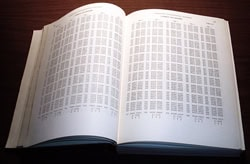Compared to your mobile phone or MP3 player, the average pocket calculator is pretty unimpressive. Sure, they make maths lessons a bit easier, but they’re hardly cutting-edge technology. But imagine if someone took away your calculator and handed you a book full of nothing but numbers as a replacement. Welcome to the world of logarithms.

### Log-a-what?

Logarithms were invented around four hundred years ago as a way of making it quicker to multiply and divide large numbers. With no calculators around, sums involving numbers of just a few digits could quickly get complicated. A Scottish mathematician named John Napier became fed up with these lengthy calculations, and created logarithms to speed things up.So what exactly is a logarithm? Think about it this way. If you’re asked “what’s 103?”, you know that the answer is 10 × 10 × 10 = 1000. Logarithms help answer a related question: “what number do I need to raise 10 by to get 1000?”. The rule is that if bx = a, then logba = x. We call “b” the base and “x” the exponent, and say that the logarithm of a to the base b is x. So the answer to our question is log101000 = 3, because the logarithm of 1000 to the base 10 is 3. The base can be any number you like, but one that crops up a lot is base 10, so we call base 10 the “common logarithm” and just write it as “log”, leaving off the 10.

### A mathematical shortcut

But how does any of this help us speed up calculations? It turns out that logarithms follow the formulas

log(u × v) = log(u) + log(v),

and

log(u / v) = log(u) – log(v).

If we know how to find the logarithm of a number, we can use these formula to turn multiplication into addition and division into subtraction.Working out logarithms requires complicated maths and can take a long time, so instead people used books of numbers, known as “logarithm tables”. Rather than work out the logarithms every time, people would rely on these printed lists of logarithms that had been calculated in advance. The tables sprang up soon after Napier invented the logarithm, and they were still in use as recently as the 1970s, before pocket calculators became cheap enough for everyone to own one.

To multiply with logarithms, you find log(u) and log(v) in the table, then add them together to get a number, which is log(u × v). You then find the antilogarithm, which tells you the answer to u × v. The number of decimal places in the tables determines how accurate the calculation is. More decimal places means greater accuracy, but also a longer and heavier book!

For example, if we want to multiply 1234 × 5678, we first find log(1234) = 3.0913 and log(5678) = 3.7542, both to five decimal places. Adding them together gives 6.8455, and the antilogarithm of 6.8455 is 7,006,500 to five significant figures. The real answer to 1234 × 5678 is 7,006,652, so even logarithm tables with just a few decimal places get us pretty close to the right answer.

### Logarithms today

Now that we have calculators you might think we don’t need logarithms any more – and you’d be wrong. It’s true that multiplication and division are no problem these days, but mathematicians, scientists, and businesses all use logarithms in another way to make numbers simpler.

When you draw a graph, you have to pick the right scale for your data. Depending on the size of the numbers, you might use 1, 10, or even 1000. If you’ve got data that uses more than one scale it can be tricky to fit it all on to one graph, but logarithms can help. On a regular scale you place the numbers 1, 2, and 3 an equal distance apart, but on a logarithmic scale you’d have the same amount of space between 1, 10, and 100. This lets you plot all the data without bunching up.

Many different things are plotted on a logarithmic scale. There’s the Richter scale, which measures the strength of earthquakes, and the decibel, which measures how loud a sound is. Financial traders need logarithms to analyse the growth of share prices, and chemists use them in the pH scale for acidity. Centuries after John Napier first invented logarithms to speed up his sums, people are still finding new uses for them – even if they get a calculator to do most of the hard work!